# Section 1 - What Will You Learn in This Tutorial?

In this tutorial, we will go over one of the advanced example model written in script and we will discuss and describe the meaning of each part of that script.

You will learn:

• Some basics about the Symbol scripting language
• How to preview a model
• How to understand the various parts of the script
• How the X-Y-Z and I-J-K Coordinates work
• How to change variables including element size
• How to display the grid lines of your model

# Section 2 - Why This Simulation?

This simple unit cell model of a single port Surface Acoustic Wave (SAW) filter allows rapid simulation of device performance. This allows a very wide range of designs to be explored before moving to more complex full 3D simulations.

The model comprises a pair aluminum electrodes on a piezoelectric substrate. The substrate can either be Lithium Tantalate (LiTaO3) or Lithium Niobate (LiNbO3). The model is set up to simulate a Y-cut, which can be rotated to the desired angle. The base design generates an shear horizontal (SH) mode at 1.5 GHz using a finger pitch of 1.3 µm.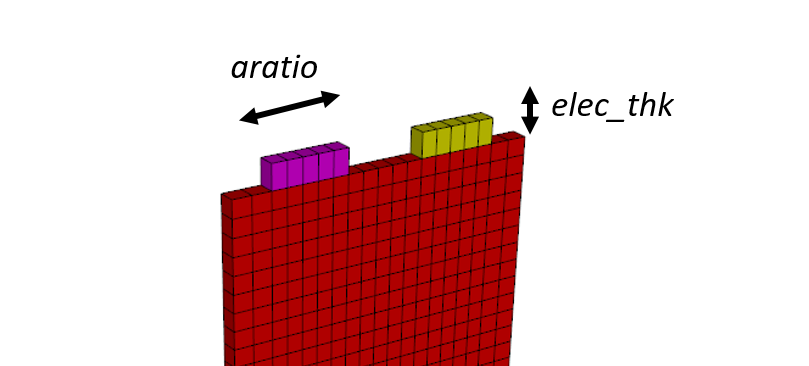This simple model represents a section of a 3D Saw filter. It is a small model with periodic boundary conditions which help a lot to calculate some critical parameters that SAW filter designers require, such as:

• S parameters
• Mode Shapes (Harmonic Analysis)
• Energy flow analysis

# Section 3 - Step by Step Video Tutorial

here is a video in which all the process of simulation has been recorded:

# Section 4 - The Tutorial Step by Step

Let's see together where to start and how to understand this tutorial

## Step 2 - Some basics about the Symbol Language

Before explaining the script model, let's talk about some of the basic functions of the "Symbol Language".

What is the symbol language? The Symbol language is a special type of script language that was developed many years ago by OnScale developers from FORTRAN when other most recent script languages weren't available. It has its own syntax which is simple to understand if you are familiar with more recent script languages such as Python or Matlab script.

### Defining a variable in the symbol language

Variables are defined in Symbol by the command symb like that:

```symb freqint = 1.5e9
symb vel = 3800.
```

After those variables have been defined, they can be used using by adding a '\$' before the name of the command, such as in the following expression, where the wavelength is calculated from the two previously defined variables

```symb wavelength = \$vel / \$freqint
```

If you put a "c" before a line of text, it means that the line of text is a comment and won't be interpreted by the solver

Comments can also be created by defining a "/*" before the text

And also, the color will change in green, like that:

```c Define frequency and meshing
symb freqint = 1.5e9					/* frequency of interest
symb vel = 3800.					/* minimum velocity
```

Those comments are very useful to understand what the script does! So pay close attention to what they say :)

These are the very basics that you need to understand in order to change a script and start playing around with parametric options, easy, right?

### How do you get a more detailed description of Symbol commands and OnScale functions that I don't understand?

If you don't understand what a command does, don't panic, just follow those 2 simple steps

1. Click on a command
2. Select Show Help for "Command Name"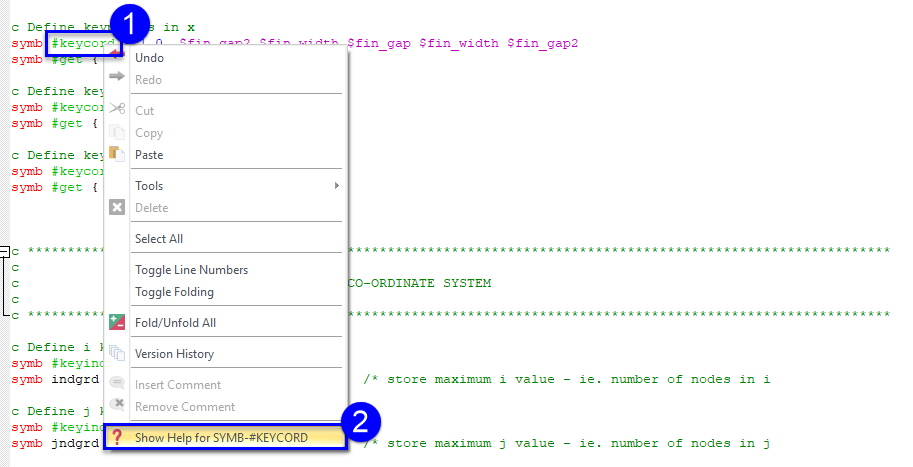The help will then be displayed with some description of what the command does and how to use it.

Note: If the Images on this page are too small, click on them to make them bigger. To close the zoom view, just click in the black unfocused area of the screen. Have a try :)

When the help appears:

1. Read carefully the description of the command
2. Try to understand the examples provided explaining how the command is used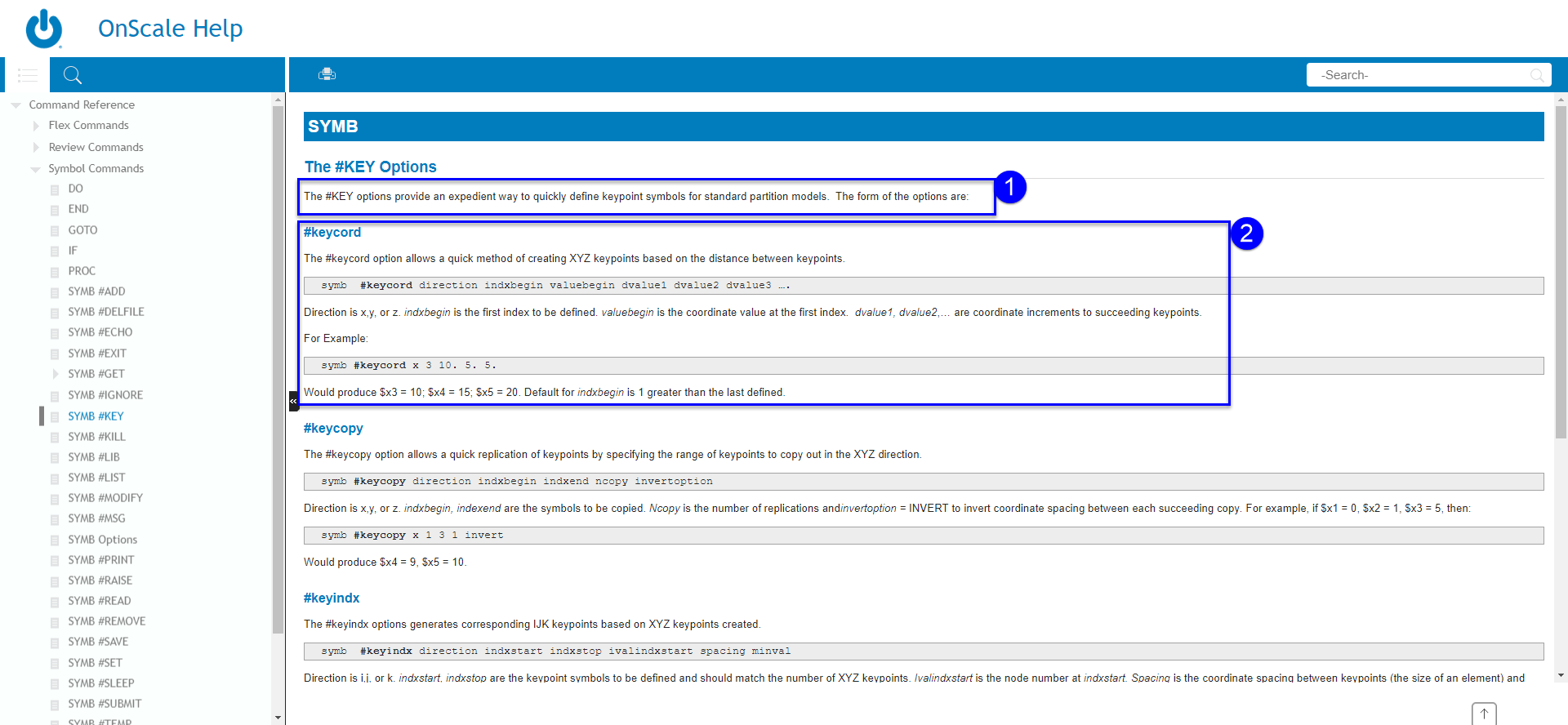## Step 3 - The opening of the script

OnScale Analyst mode script always starts with the following few commands.

What they do is self-explained in the comment green description

```c The adds a title and description to output files
titl  saw_unit_3D	3D Unit Cell Model of SAW Filter

c Use all cores on machine
mp omp * *

c No restart file
rest no
```

## Step 4 - Defining the Model Parameters

Every OnScale Analyst Model is created by defining a few key variables... and by using those variables that OnScale is able to turn your model into a parametric model.

If you only use the Designer Mode, you don't see those parameters, but that's only because they are defined inside the analyst script generated by the designer model that you only see if you open that script (this script is always saved with the extension *.flxinp)

In this specific model, this is how they have been defined:

```c Thicknesses
symb subs_thk = 7.5e-6					/* substrate thickness
symbx elec_thk = 200.e-9				/* electrode thickness

c IDT
symb fin_pitch = 1.30e-6				/* finger pitch
symbx aratio = 0.60					/* metalization aspect ratio
symb fin_width = \$fin_pitch * \$aratio	                /* finger width
symb fin_gap = \$fin_pitch - \$fin_width	                /* finger gap
symb nfing = 100					/* pairs
symb fin_len = 50.e-6					/* finger length

c Active material
text piezo_mat = 'lt'	/* piezo material: 'lt' for LiTaO3, or 'lno' for LiNb03
symb cut_ang = 42.	/* rotated y-cut angle

c Calculations
symb ascal = \$nfing * \$fin_len / \$box
symb fin_width2 = \$fin_width / 2.
symb fin_gap2 = \$fin_gap / 2.
symb fin_len2 = \$fin_len / 2.
symb fin_pitch = ( \$fin_width + \$fin_gap ) * 2.
symb simtime = \$ncycles / \$freqint		/* total runtime of the model
text piezo_struc = '\$(piezo_mat)struc'

```

Those are the variables which define the geometry of the model: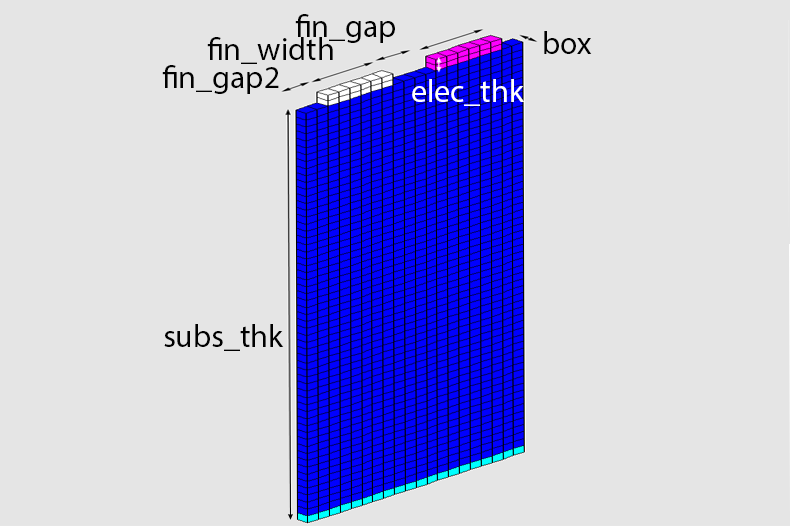You then have other variables which are used to defined a bunch of other parameters that you will need in your simulation:

```c Define frequency and meshing
symb freqint = 1.5e9			/* frequency of interest
symb vel = 3800.			/* minimum velocity
symb nelem = 20				/* number of elements per wavelength
symb wavelength = \$vel / \$freqint	/* calculate wavelength
symb box = \$wavelength / \$nelem		/* calculate box size for model
symb freqdamp = \$freqint		/* damping frequency, used in damping models

c Runtime
symb ncycles = 2500			/* run model for N cycles
symb nloops = 10			/* plot model to screen this many times
symb tfact = 0.80			/* set time stability factor - default 0.8

c EBond
symb nbondopt = 1			/* activate periodic electric boundaries

```

In the above code snippet the frequency and mesh settings are used to define the mesh box size for more information on this refer to the linked article:

Model Inputs & Meshing

## Step 5 - Defining the X-Y-Z and I-J-K Coordinate Systems

In OnScale, you have 2 coordinate systems:

• The X-Y-Z Coordinate System which provides the size of the geometry
• The I-J-K Coordinate System which provides the size of the grid

You can understand it like this:

The X-Y-Z Coordinate System is where the physical geometry dimensions of your model reside, whereas the I-J-K Coordinate System is where the associated Finite Element Grid is created.

This is how the X-Y-Z Coordinate System definition looks:

```c Define keypoints in x
symb #keycord x 1 0. \$fin_gap2 \$fin_width \$fin_gap \$fin_width \$fin_gap2
symb #get { idx } rootmax x

c Define keypoints in y
symb #keycord y 1 0. \$box
symb #get { jdx } rootmax y

c Define keypoints in z
symb #keycord z 1 0. \$subs_thk \$elec_thk
symb #get { kdx } rootmax z	```

This is how the I-J-K Coordinate System definition looks:

```c Define i keypoints
symb #keyindx i 1 \$idx 1 \$box
symb indgrd = \$i\$idx		/* store maximum i value - ie. number of nodes in i

c Define j keypoints
symb #keyindx j 1 \$jdx 1 \$box
symb jndgrd = \$j\$jdx		/* store maximum j value - ie. number of nodes in j

c Define k keypoints
symb #keyindx k 1 \$kdx 1 \$box
symb kndgrd = \$k\$kdx		/* store maximum j value - ie. number of nodes in j

c List symbols
symb #list
```

We have used some simple calculations that can be seen on lines 116-129 of the script that calculates nodes/elemnts/DOF and outputs them to the console once the model is running

## Step 6 - Grid and geometry definition

The following two commands are required and must be used in in every model being scripted. The grid command calculates the number of nodes in the grid, the number of dimensions for the model and can also be used to define appropriate constraint relations if applicable. The total number of nodes is calculated by multiplying the last node indices of the I-J-K coordinate system, from the previous step the last node is stored in the variables being passed into the grid command.

`c Create gridgrid \$indgrd \$jndgrd \$kndgrd`

Geom is used to define the nodal coordinates of the grid.

`c Assign geometry, shortened versiongeom keypnt \$idx \$jdx \$kdx`

## Step 7 - Materials definition and assignment

An important aspect, which is also important in designer. Is the proper use of materials as improper use of materials will lead to inaccurate results. We have a small material database that you can use but we recommend that you characterise the materials manually for more information on this refer to the linked article:

Materials & Geometry

To complete the geometry setup, lastly we need to assign materials to appropriate region the grid. This is made easier through the creation of the keypoints in step 5.

`c Read material filesymb #read saw.prjmatc Assign materials to gridsite     /* Void all of grid     regn void            /* initialise all elements by assigning void material      /* Layers     regn \$piezo_mat * * * * \$k1 \$k2      regn \$piezo_struc * * * * \$k1 \$k1+1     /* Electrodes     regn alum1 \$i2 \$i3 * * \$k2 \$k3      regn alum2 \$i4 \$i5 * * \$k2 \$k3      end`

The use of * * means that the material is assigned from the first keypoint to the last keypoint in that direction. As mentioned in Step 2 have a look at the command reference for the site commands to see what each individual parameter being passed into the sub-command is used for. The parameter \$k1+1 adds 1 node to the keypoint k1 so this defines a thin strip of structural material that we have used in this simulation.

## Step 8 - Boundary Conditions

Now with materials having been fully assigned to the grid, external boundaries of the device need to be set up correctly these will reflect how the areas beyond the model would behave in the real world.

We have a number of predefined boundaries the most common are free, fixed (fixd), absorbing (absr) and symmetry (symm). This example uses a more advanced boundary condition, the periodic boundary condition and there are extra steps needed in order to use a periodic boundary condition correctly.

`c Define external boundary conditionsboun     side zmin absr     side zmax free     endc Periodic boundariesbond       defn bperx nchk       cors \$i1 \$i1 * * * *       fine \$indgrd \$indgrd * * * *        defn bpery nchk       cors * * \$j1 \$j1 * *       fine * * \$j2 \$j2 * *       end`

Normally boundary conditions would be defined using the BOUN command but because we are using periodic boundaries as these are more accurate when simulating the unit cell as it assumes a large (infinite) system, we need to use the BOND command to apply a periodic boundary condition. This will bond the surface of one side to the other it must be defined as a region (made easier with keypoints) and the sides must be parallel to each other.

## Step 9 - Defining drive function

Before we set up the loading conditions, an input waveform, also referred to as a Time Function, is required. There are two ways to do this - manually or using the Time Function tool which provides a visual representation of the waveform and provides a syntax that can be copy and pasted into the script. We have done it manually. We have driven the unit cell with a ricker wavelet centred at 1.5GHz at a 1V amplitude. OnScale recommends using the Ricker Wavelet (wvlt) time function due to its broadband performance and low spectral leakage beyond 2.5x the centre frequency.

`c Define drive function, can be accessed using 'func' argumentfunc wvlt \$freqint 1.`

## Step 10 - Creating electrodes

Defining the piezoelectric load requires the PIEZ command. A piezoelectric window is defined this defines the area of the grid designated for the electro-mechanical calculation. Periodic boundaries must also be defined here so that this is taken into consideration when the electro-mechanical solve is being carried out. The solver is also chosen for the electrostatic solve, pard is typically the fastest especially for large piezoelectric models.

`c Define piezo solvepiez     /* Define electric window     wndo * * * * \$k1 \$k2       /* IDT Inner     defn idt1 \$ascal     node \$i2 \$i3 * * \$k2 \$k2     bc idt1 volt func     /* IDT Outer     defn idt2 \$ascal     node \$i4 \$i5 * * \$k2 \$k2     bc idt2 grnd     side 1 periodic     side 3 periodic      /* Set solver     slvr pard     end`

The two electrodes defined lie on the surface encapsulated by the nodes in the below image.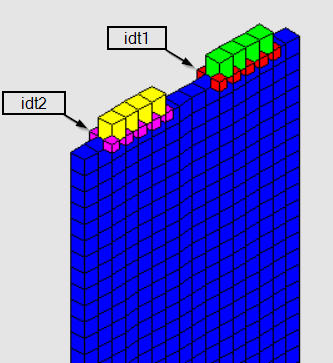## Step 11 - Outputs

Time Histories in OnScale are simply data fields captured over time for specific nodes/elements in the model. As a time-domain solver, OnScale can generate any of the calculated data arrays as a set of time histories. The below lines of code output the drive function connected to idt1 and the voltage and charge on that electrode you can also output current by changing 'vq' to vqi' change or copy the line to output the same information for idt2.

Mode shapes are extracted through Harmonic Analysis methods. We extract the harmonic behaviour of a system by performing DFTs at specified frequencies. This can also be performed for specified data fields of interest such as displacements. For more information on mode shapes refer to the linked article:

Mode Shape Normalisation

`c Time histories to storepout     hist func                        /* #1 Drive function     histname electrode vq idt1       /* #2 - #5 Voltage and charge on each electrode     endc Mode shapesshap     twnd \$simtime hann righ 1.     freq \$freqint     data ydsp     end`

## Step 12 - Model execution and extracting outputs

Before running the model you should always issue the PRCS command. It computes the model time step, this is for stability and also opens necessary arrays for storage. This is very easy see below, for more information on time-step and time stability factor refer to the linked article:

Is there anyway to speed-up my model?

`c Time stability factortime * * \$tfactc Process model, including setting time stepprcs `

Lets start looking at model execution. Needed is model timestep, simulation time and the simulation time in terms of timesteps. We already defined the simulation time and the timestep is calculated when you call to prcs, we just need to store this in a variable and use it to calculate the simulation time in terms of timesteps (nexec). Nexec2 is used for the runtime graphics loop that we will touch on shortly

`c Set up run parameterssymb #get { step } timestep          /* store timestep size in variable 'step'symb nexec = \$simtime / \$step        /* simulation time in terms of timestepssymb nexec2 = \$nexec / \$nloops       /* number of executions in a loop`

Before the execution loop we will set up plots:

`c Set up graphicsgrph     nvew 2 2 /* two plotting windows     end`

This is the optimal way of creating an execution loop. It is similar to a do loop, without using a do loop. The procedure is a part of the code that can be recalled a number of times using the PROC command. This is where we use 'nexec2' which is our simulation time in time steps divided by the number of loops you want to see, simple.

`c Define run/plot procedureproc plot savec Run model for some timeexec \$nexec2c Plot current state of modelgrph     plot yvel       /* plot acoustic velocity in y     plot 3          /* plot charge on top electrode     endend\$ proc            /* end of proc`

Last and most importantly outputting the results. After the simulation has fully executed, we can ask the solver to output certain data calculated during the simulation to an output file (.flxdato) ready to be post-processed. More information can be found in the linked article:

Model Outputs

This is very simple see below:

`c Output shape datadata    out modl         /* Outputs model geometry     out shap/all     /* Outputs all requested mode shapes    end`

It is also good modelling practice to output all the symbol variables used in the model. This gives the user insight into settings used or perhaps to allow the variables to be read into a post processing script, we haven't done this in the file attached but feel free to add this in yourselves :)

`c save symbols to file for later usesymb #get { label } jobname          /* get model job namesymb #save \$label.symb               /* save symbol filec end of input file     stop`

## Step 13 - Running the model and post processing results

Running the model on the cloud is incredibly easy simple open the cloud scheduler and click the estimate button. After the estimation is complete you simply click run and your simulation files will be uploaded to the cloud.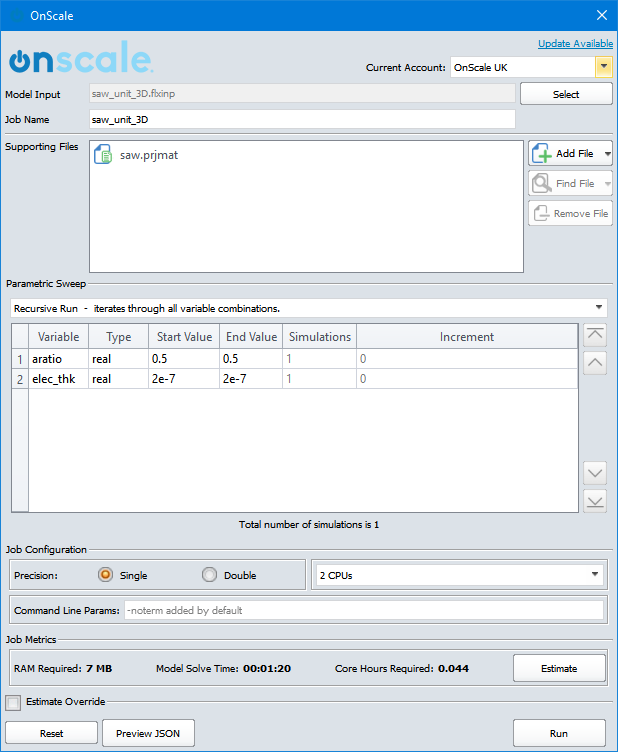Once the simulation is complete, access the cloud storage download the results and you are now ready to post process the results.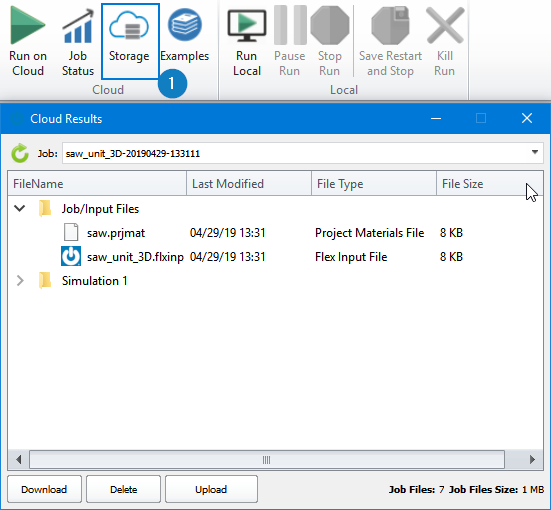We will make a switch to the post processor to do this. This can be done by opening up another OnScale application window and choosing the post processor from the 3 options or a quick switch can be made by clicking the icon found in the top right hand corner of the application.

The two output file types generated are the flxhst and flxdato files. flxhst store all time history records generated and flxdato files store all the data arrays/mode shapes requested.

Here we can calculate the impedance and admittance refer to the linked video to see how to extract Electrical Impedance, this process is exactly the same for Admittance the option to calculate Admittance is under Impedance!:

Extracting Electrical Impedance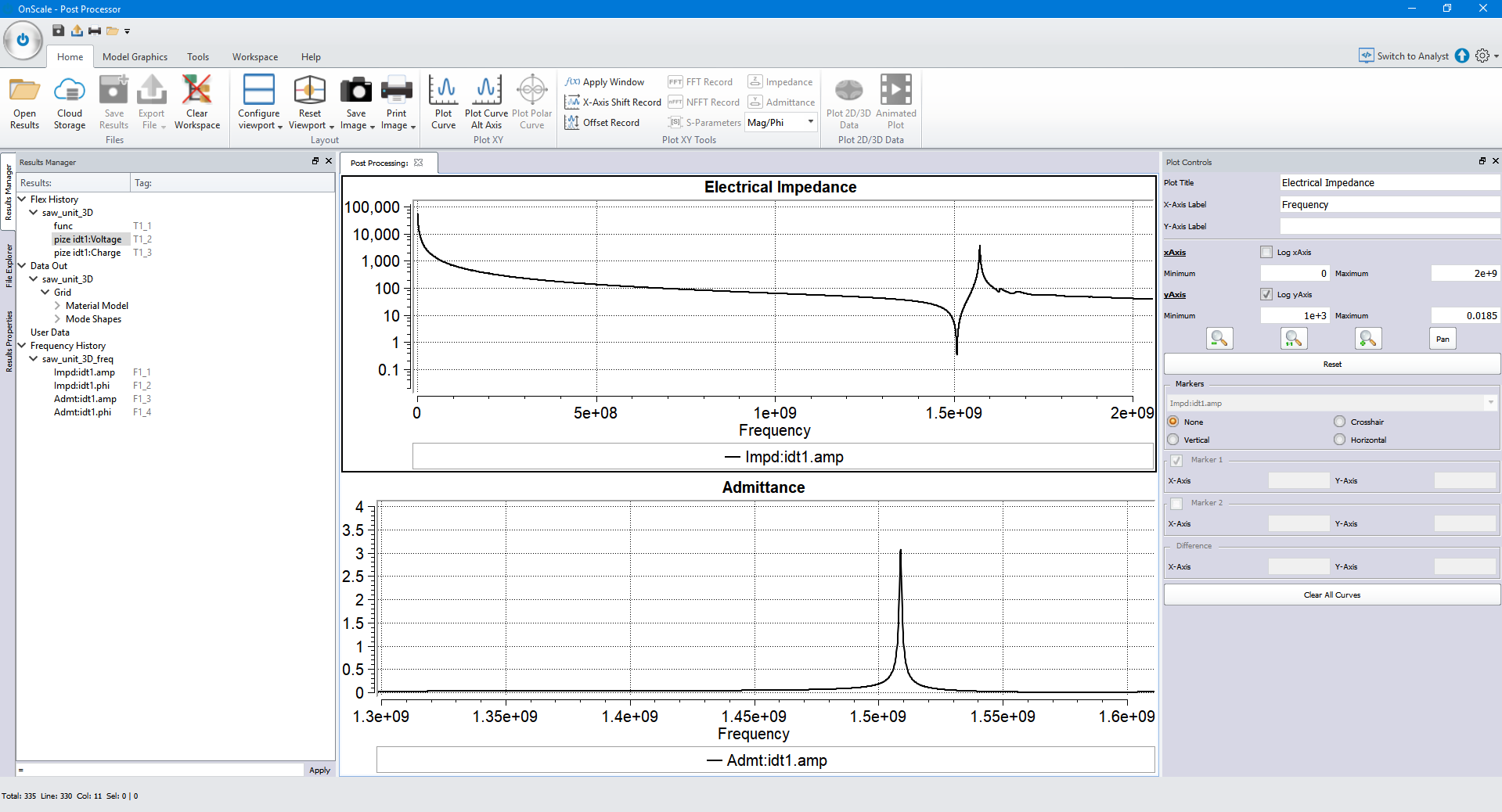Similarly we extracted a mode shape of Y-displacement this can be plotted as an animated plot, see the video linked for a detailed demonstration of how to plot and view mode shapes:

Extracting and Viewing Mode Shapes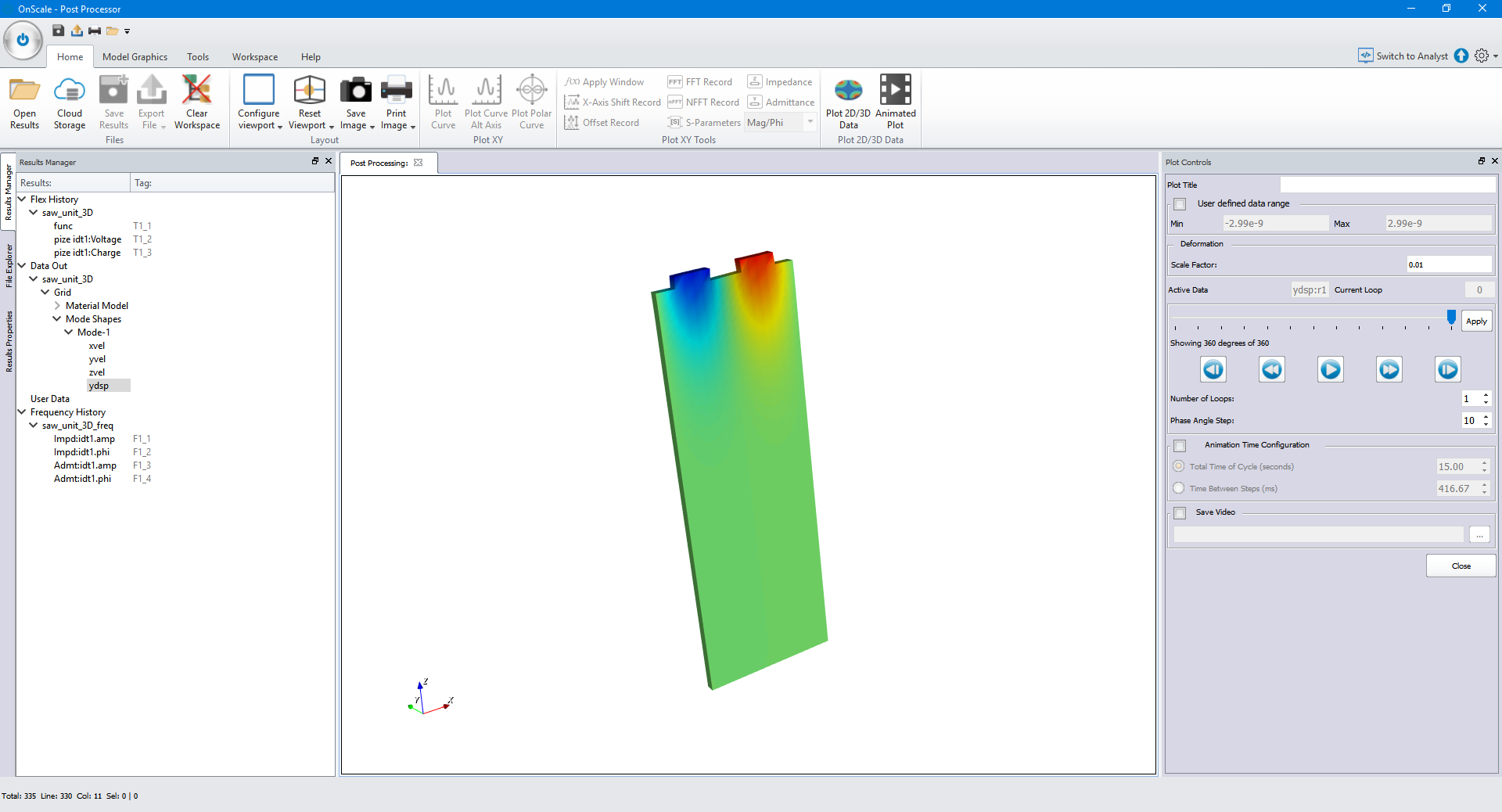0 out of 0 found this helpful